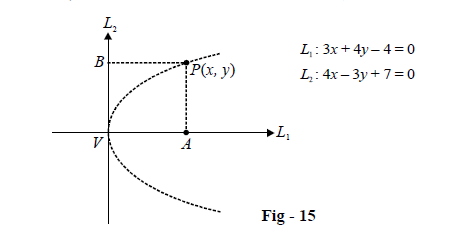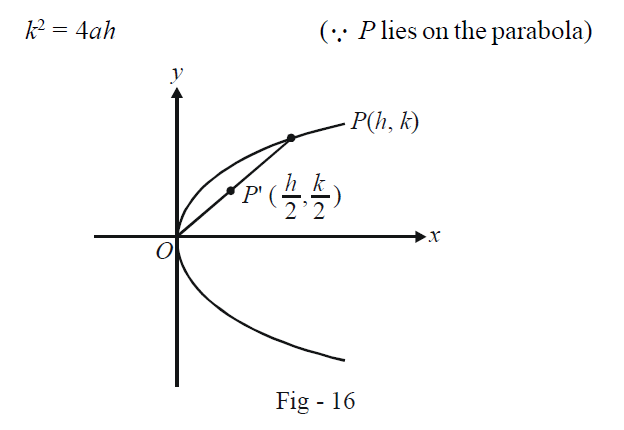# Basic Examples on Parabolas Set 1

Go back to  'Parabola'

Example - 3

Find the length of the LR of the parabola $$4{y^2} + 12x - 12y + 39 = 0$$

Solution: The equation can be rearranged as (verify)

\begin{align}&\qquad \;\;{\left( {y - \frac{3}{2}} \right)^2} = - 3\left( {x + \frac{5}{2}} \right)\\&\Rightarrow \quad {\left( {y - \frac{3}{2}} \right)^2} = - 4 \times \left( {\frac{3}{4}} \right) \times \left( {x + \frac{5}{2}} \right)\end{align}

This is of the form

${Y^2} = - 4aX{\rm{ }}\left( {a = \frac{3}{4}} \right)$

i.e. this is a parabola with vertex \left(\begin{align} {\frac{3}{2},\,\,\frac{5}{2}}\end{align} \right) and axis parallel to the x-axis. The parabola opens to the left. We are only concerned with the length of the LR which is simply 4a, i.e. 3 units.

Example – 4

The axis of a parabola is the line $${L_1}:3x + 4y - 4 = 0$$ and the tangent to it at the vertex is $${L_2}:4x - 3y + 7 = 0.$$ The LR is 4 units in length. Find the equation of the parabola.

Solution: Consider for a moment, the co-ordinate axes system formed by $${L_1}and{\rm{ }}{L_2}$$.If $${L_1}\;and\; {\rm{ }}{L_2}$$ were truly the actual co-ordinate axes, the equation of the parabola would have been

\begin{align}&\qquad \;\;{y^2} = 4ax\\\\&\Rightarrow \quad P{A^2} = ({\rm{Length\;of\; }}LR) \times PB...\left( 1 \right)\end{align}

Now, even if we use some other co-ordinate system (here the $${L_1} - {\rm{ }}{L_2}$$ system), the relation (1) will still hold since that only depends on lengths which are invariant with respect to the co-ordinate system chosen. Thus, simply applying (1) will give us the required equation :

\begin{align}& \qquad\;{\left( {\frac{{\left| {3x + 4y - 4} \right|}}{5}} \right)^2} = (4) \times \frac{{\left| {4x - 3y + 7} \right|}}{5}\\&\Rightarrow \quad {(3x + 4y - 4)^2} = \pm 20(4x - 3y + 7)\end{align}

Thus, as might have been expected, we’ll obtain two parabolas with the given property, one opening to the ‘left’ and one to the ‘right’ in the $${L_1} - {\rm{ }}{L_2}$$ co-ordinate system.

Example - 5

Show that the locus of the middle points of all chords of the parabola $${y^2} = 4ax$$ which pass through the origin, is another parabola.

Solution: Let OP be any such chord of the parabola, where O is the origin (0, 0) and P is the point (h, k) lying somewhere on the parabola. We then haveThe mid-point of OP, say P' is clearly \left( \begin{align}{\frac{h}{2},\,\,\frac{k}{2}} \end{align}\right) .

Since $${k^2} = 4ah,$$ we have

\begin{align}&\qquad {\left( {\frac{k}{2}} \right)^2} = 2a\left( {\frac{h}{2}} \right)\\\\&\Rightarrow \quad {y^2} = 2ax\qquad\qquad\qquad...\left( 1 \right)\end{align}

where we used (x, y) instead of \left(\begin{align} {\frac{h}{2},\,\,\frac{k}{2}} \end{align}\right) to specify the equation of the locus of P' in the conventional x – y form.

(1) shows that the required locus is another parabola, which has the same vertex and axis.

Download SOLVED Practice Questions of Basic Examples on Parabolas Set 1 for FREE
Parabolas
grade 11 | Questions Set 1
Parabolas
grade 11 | Answers Set 1
Parabolas
grade 11 | Questions Set 2
Parabolas
grade 11 | Answers Set 2
Learn from the best math teachers and top your exams

• Live one on one classroom and doubt clearing
• Practice worksheets in and after class for conceptual clarity
• Personalized curriculum to keep up with school